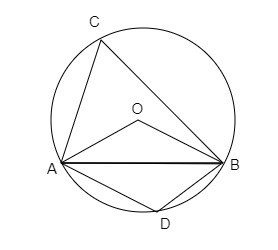# A chord of a circle is equal to the radius of the circle. Find the angle subtended by the chord at a point on the minor arc and also at a point on the major arc.

Given:

A chord of a circle is equal to the radius of the circle.

To do:

We have to find the angle subtended by the chord at a point on the minor arc and also at a point on the major arc.

Solution:In the figure, AB is equal to the radius of the circle.

In $\triangle OAB$,

$OA=OB=AB$    (Radii of the circle)

Therefore,

$\triangle OAB$ is an equilateral triangle.

$\angle AOC=60^o$

$\angle ∠ACB= \frac{1}{2}\angle AOB=\frac{1}{2}\times60^o=30^o$

This implies,

$\angle ACB+\angle ADB=180^o$       (Opposite angles of a cyclic quadrilateral are supplementary)

$\angle ADB=180^o−30^o=150^o$.

Therefore, angles subtended by the chord at a point on the minor arc and at a point on the major arc are $150^o$ and $30^o$ respectively.

Updated on: 10-Oct-2022

65 Views